# FPGA-Based Beamforming in Simulink: Algorithm Design

This example shows the first half of a workflow to develop a beamformer in Simulink® suitable for implementation on hardware, such as a field programmable gate array (FPGA). It also shows how to compare the results of the implementation model with those of a behavioral model.

The second part of the example FPGA-Based Beamforming in Simulink: Code Generation shows how to generate HDL code from the implementation model and verify that the generated HDL code produces the correct results compared to the behavioral model.

This example shows how to implement an FPGA-ready beamformer to match a corresponding behavioral model in Simulink by using the Phased Array System Toolbox™, DSP System Toolbox™, and Fixed-Point Designer™ libraries. To verify the implementation model, this example compares the simulation output of the implementation model with the output of the behavioral model.

The Phased Array System Toolbox is used to design and verify the floating-point functional algorithm, which provides the behavioral reference model. The behavioral model is then used to verify the results of the fixed-point implementation model used to generate HDL code.

Fixed-Point Designer provides data types and tools for developing fixed-point and single-precision algorithms to optimize performance on embedded hardware. You can perform bit-true simulations to observe the impact of limited range and precision without implementing the design on hardware.

### Partitioning Model for FPGA

There are three key modeling concepts to keep in mind when preparing a Simulink model to target FPGAs:

• Sample-based processing: Also commonly referred to as serial processing, sample-based processing is an efficient data processing technique in hardware designs that enables you to tradeoff between resources and throughput.

• Subsystem targeted for HDL code generation: In order to generate HDL code from a model, the implementation algorithm must be inside a Simulink subsystem.

• Time-aligned outputs of behavioral and implementation models: For comparing the outputs of the behavioral and FPGA implementation models, you must time align their outputs by adding latency to the behavioral model.

### Beamforming Algorithm

This example shows a phase-shift beamformer as the behavioral algorithm, which is re-implemented in the `HDL Algorithm` subsystem by using Simulink blocks that support HDL code generation. The beamformer calculates the phase required between each of the ten channels to maximize the received signal power in the direction of the incident angle. The figure shows the Simulink model with the behavioral algorithm and its corresponding implementation algorithm for an FPGA.

```modelname = 'SimulinkBeamformingHDLWorkflowExample'; open_system(modelname); set(allchild(0),'Visible','off'); ```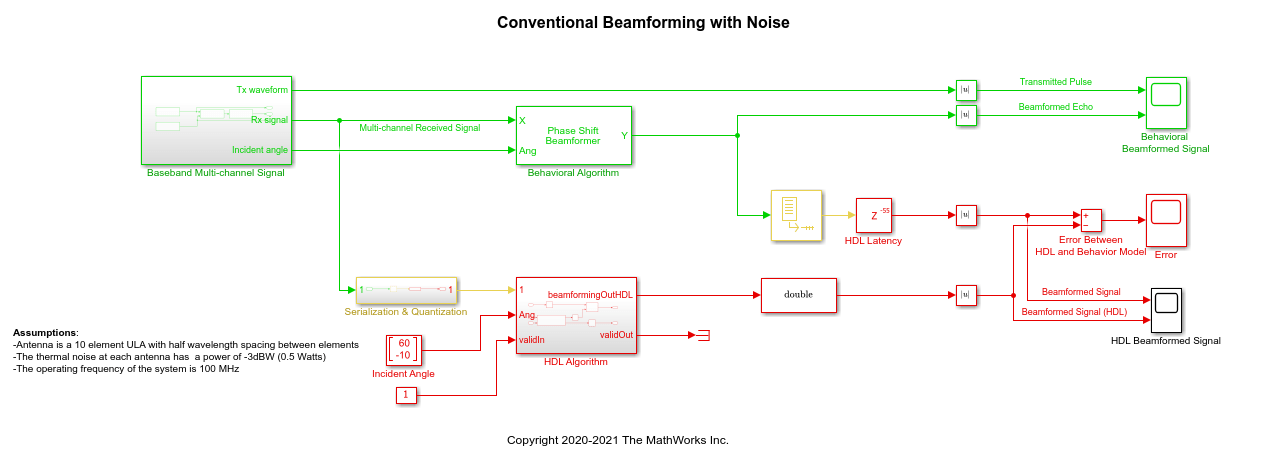The Simulink model has two branches. The top branch is the behavioral, floating-point model of the algorithm and the bottom branch is the functionally-equivalent fixed-point version using blocks that support HDL code generation. Besides plotting the output of both branches to compare the two, this example also calculates and plots the difference, or error, between both outputs.

The model has a Delay (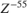) block at the output of the behavioral algorithm. This delay is necessary because the implementation algorithm uses 55 delays to implement pipelining which creates latency that needs to be accounted for. Accounting for this latency is called delay balancing and is necessary to time-align the output between the behavioral model and the implementation model to make it easier to compare the results.

To synthesize a received signal at the phased array antenna, the model includes a subsystem that generates a multi-channel signal. The `Baseband Multi-channel Signal` subsystem models a transmitted waveform and the received target echo at the incident angle captured via a 10-element antenna array. The subsystem also includes a receiver pre-amp model to account for receiver noise. This subsystem generates the input stimulus for our behavioral and implementation models.

```open_system([modelname '/Baseband Multi-channel Signal']); ```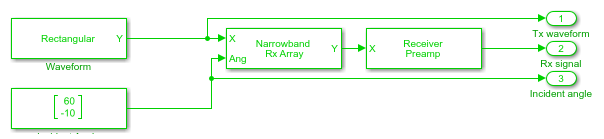### Serialization and Quantization

The model includes a `Serialization & Quantization` subsystem which converts floating-point, frame-based signals to fixed-point, sample-based signals necessary for modeling streaming data in hardware. This model uses scalar streaming processing because this algorithm runs slower than 400 MHz. The hardware implementation can be optimized for resources rather than for higher throughput.

```open_system([modelname '/Serialization & Quantization']); set_param(modelname,'SimulationCommand','update') ```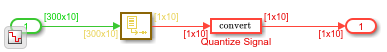The input signal to the serialization subsystem has 10 channels with 300 samples per channel or a 300x10 size signal. The subsystem serializes, or unbuffers, the signal producing a sample-based signal that's 1x10, i.e., one sample per channel, which is then quantized to meet the requirements of the system.

The output data type of the Quantize Signal block is `fixdt(1,12,9)`, which is a signed value with 12-bit word length and 9-bit fraction length precision. The word length is 12 bits because the design is targeted to a Xilinx® Virtex®-7 FPGA which is connected to a 12-bit ADC. The fraction length accommodates the maximum range of the input signal.

### Designing the Implementation Subsystem

The `HDL Algorithm` subsystem, which is targeted for HDL code generation, implements the beamformer, which was designed using Simulink blocks that support HDL code generation.

The `Angle2SteeringVec` subsystem calculates the signal delay at each antenna element of a Uniform Linear Array (ULA). The delay is then fed to a multiply and accumulate (MAC) subsystem to perform beamforming.

```open_system([modelname '/HDL Algorithm']); ```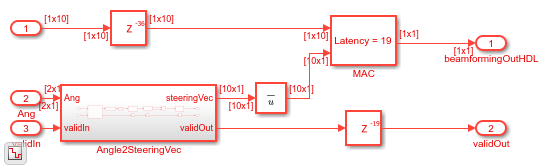The algorithm in the `HDL Algorithm` subsystem is functionally equivalent to the phase-shift beamforming behavioral algorithm but can generate HDL code. There are three main differences that enable this subsystem to generate efficient HDL code.

1. The design processes inputs serially, i.e. using sample-based processing.

2. The subsystem uses fixed-point data types for any calculations.

3. The implementation includes Delays that enable pipelining by the HDL synthesis tool.

To ensure proper clock timing, any delay added to one branch of the implementation model must be matched to all other parallel branches as seen above. The `Angle2SteeringVec` subsystem, for example, has 36 delays; therefore, the top branch of the `HDL Algorithm` subsystem includes a delay of 36 samples right before the `MAC` subsystem. Likewise, the `MAC` subsystem uses 19 delays, which must be balanced by adding 19 delays to the output of the `Angle2SteeringVec` subsystem. This figure shows the inside of the `MAC` subsystem to illustrate the 19 delays.

```open_system([modelname '/HDL Algorithm/MAC']); set_param(modelname,'SimulationCommand','update') ```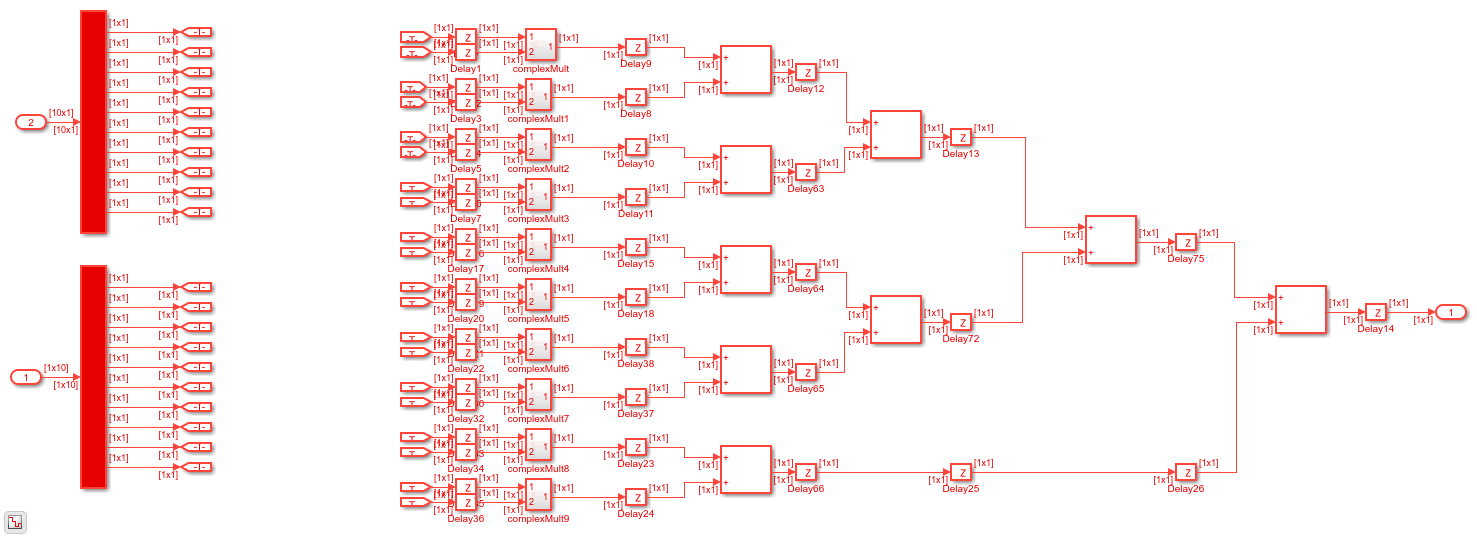The bottom branch of the `MAC` subsystem has a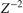block, followed by the complex multiply block which contains a, then a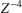block, followed by 4 delay blocks of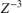for a total of 19 delays. The delay values are defined in the PreLoadFcn callback in Model Properties.

### Calculating the Steering Vector

The `Angle2SteeringVec` subsystem calculates the steering vector from the signal's angle of arrival. It first calculates the signal's arrival delay at each sensor by matrix-multiplying the antenna element position in the array by the incident direction of the signal. The delays are then passed to the `SinCos` subsystem which calculates the sine and cosine trigonometric functions by using the simple and efficient CORDIC algorithm.

```open_system([modelname '/HDL Algorithm/Angle2SteeringVec']); ```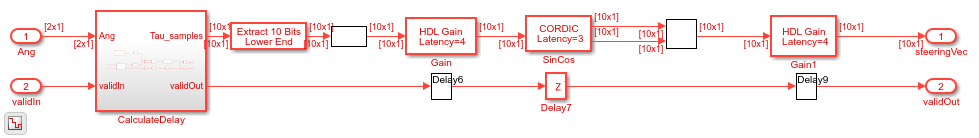Because this design consists of a 10 element ULA spaced at half-wavelength, the antenna element position is based on the spacing between each antenna element measured outwardly from the center of the antenna array. Define the spacing between elements as a vector of 10 numbers ranging from -6.7453 to 6.7453, i.e., with a spacing of 1/2 wavelength, which is 2.99/2. In fixed-point arithmetic, the data type used for the element spacing vector is `fixdt(1,8,4)`, i.e., a signed value with 8-bit word length and 4-bit fraction length.

### Deserialization

To compare your sample-based fixed-point implementation with the floating-point frame-based behavioral design you need to deserialize the output of the implementation subsystem and convert it to a floating-point data type. Alternatively, you can compare the results directly with sample-based signals but then you must unbuffer the output of the behavioral model to match the sample-based signal output from the implementation algorithm, as shown in this figure.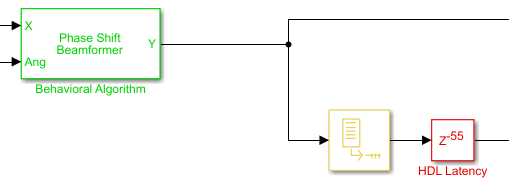In this case, you only need to convert the output of the `HDL Algorithm` subsystem to floating-point by setting the output of the Data Type Conversion block to `double`.

### Comparing Output of HDL Model to Behavioral Model

Run the model to display the results. You can run the Simulink model by clicking the Play button or calling the sim command from the MATLAB® command line. Use the scopes to compare the outputs visually.

```sim(modelname); ```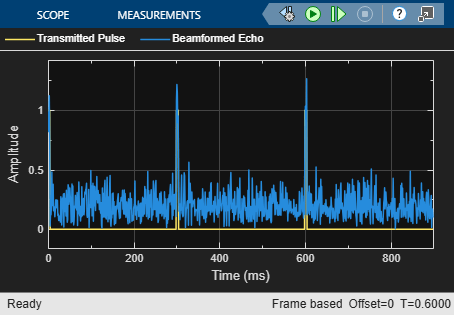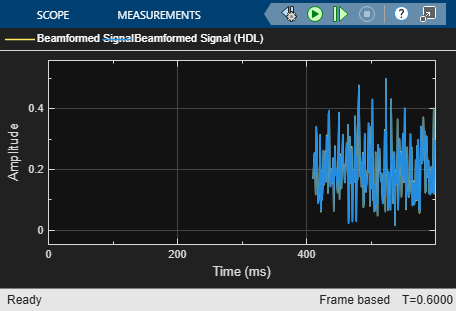The Time Scope of the Beamformed Signal and Beamformed Signal (HDL) shows that the two signals are nearly identical. The error is on the order of 10^-3 in the Error scope. This result shows that the `HDL Algorithm` subsystem is producing the same output as the behavioral model within quantization error. This verification is an important first step before generating HDL code.

Because the HDL model used 55 delays, the scope titled HDL Beamformed Signal is delayed by 55ms when compared to the original transmitted or beamformed signal shown on the Behavioral Beamformed Signal scope.

### Summary

This example is the first of a two-part tutorial series on how to design an FPGA-ready algorithm, automatically generate HDL code, and verify the HDL code in Simulink. This example showed how to use blocks from the Phased Array System Toolbox to create a behavioral model to serve as a golden reference, and how to create a subsystem for hardware implementation using Simulink blocks that support HDL code generation. It also compared the output of the implementation model to the output of the corresponding behavioral model to verify that the two algorithms are functionally equivalent.

Once you verify that your implementation algorithm is functionally equivalent to your golden reference, you can use HDL Coder™ for HDL Code Generation from Simulink (HDL Coder) and HDL Verifier™ to Generate a Cosimulation Model (HDL Coder) test bench.

The second part of this two-part tutorial series FPGA-Based Beamforming in Simulink: Code Generation shows how to generate HDL code from the implementation model and verify that the generated HDL code produces the same results as the floating-point behavioral model as well as the fixed-point implementation model.